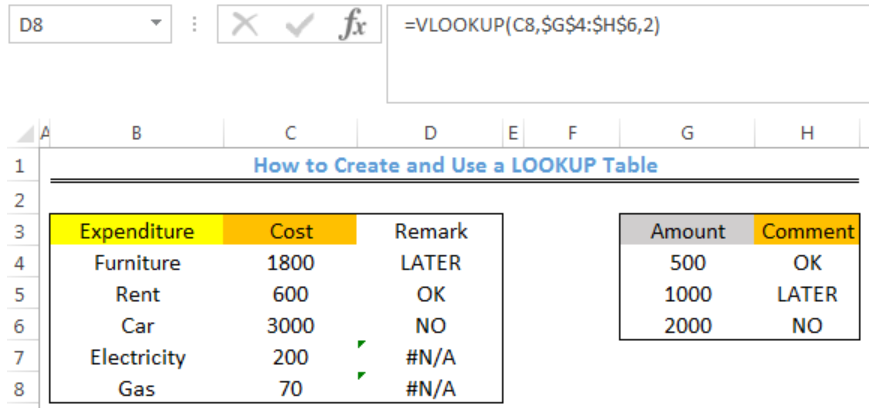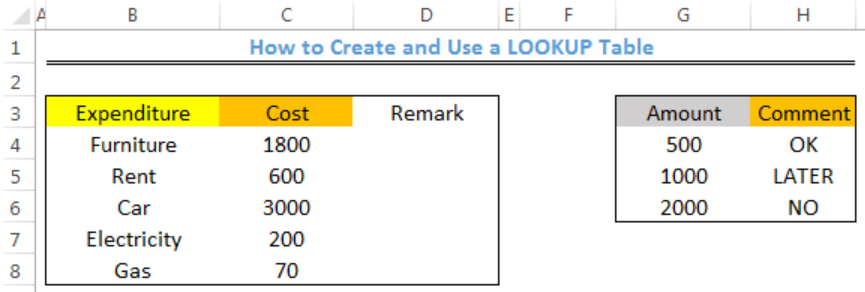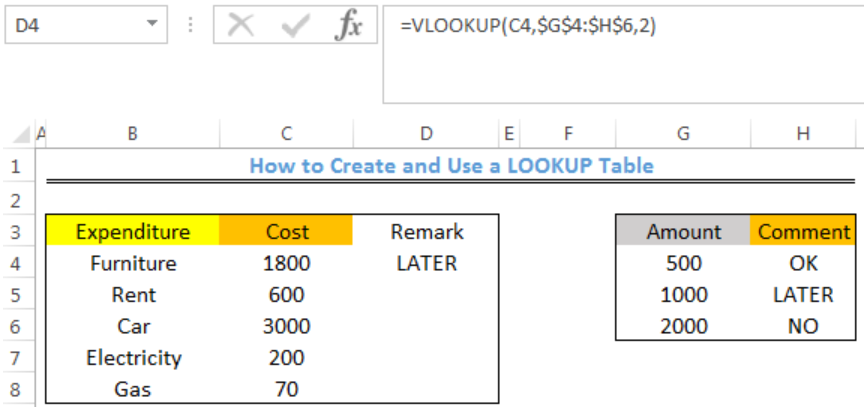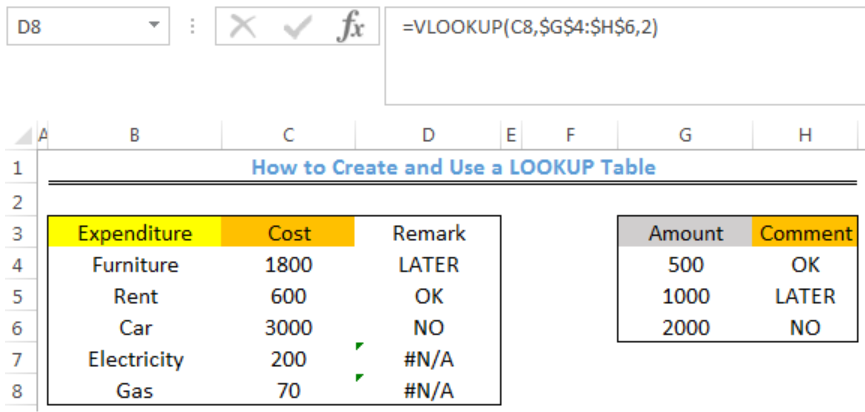Get instant live expert help with Excel or Google Sheets“My Excelchat expert helped me in less than 20 minutes, saving me what would have been 5 hours of work!”

#### Post your problem and you’ll get expert help in seconds.

Your message must be at least 40 characters
Our professional experts are available now. Your privacy is guaranteed.

# How to Create and Use a LOOKUP Table in Excel

We can create and use a LOOKUP TABLE in excel for sorting large amount of data. The LOOKUP TABLE allows us to evaluate cells and input an associated comment or remark. The steps below will walk through the process.Figure 1- How to Create and Use a LOOKUP Table in Excel

## Syntax

`=VLOOKUP(lookup_value,table_array,col_index_num,[range_lookup])`

• Lookup_value: This is the value to search for
• Table_array: This is the range to search for the lookup value
• Col_index_num: This number specifies the column where we want the value to be returned from
• Range_lookup: This is used to specify if we want and approximate or exact match of the lookup value. If omitted, VLOOKUP assumes an approximate match

## Formula

`=VLOOKUP(C4,\$G\$4:\$H\$6,2)`

## Setting up the Data

We will use the LOOKUP TABLE to ascertain based on the comment in Column H if we will proceed with the stated expenditure in COLUMN C as shown in figure 2.

• We will enter the expenditure into Column B
• We will enter the cost into Column C
• Column D contains the Remarks where the results will be returned by VLOOKUPFigure 2 – Setting up the Data

## Using the VLOOKUP Function in the LOOKUP TABLE

• We will click on Cell D4
• We will insert the formula below into Cell D4
`=VLOOKUP(C4,\$G\$4:\$H\$6,2)`
• We will press the enter keyFigure 3- VLOOKUP result for Remarks about the Furniture Expenditure

• We will click on Cell D4 again
• We will double click on the fill handle tool which is the small plus sign you see at the bottom right of Cell D4. Select and drag down to copy the formula to Cell D8Figure 4- VLOOKUP results for Remarks about all Expenditure

## Note

• The VLOOKUP function assumes that the lookup table is sorted in ascending order
• If the lookup_value is greater than every value in the lookup table, the LOOKUP function matches the last value
• If the lookup_value is less than all values in the lookup table, the VLOOKUP function returns the #N/A error

## Instant Connection to an Expert through our Excelchat Service

Most of the time, the problem you will need to solve will be more complex than a simple application of a formula or function. If you want to save hours of research and frustration, try our live Excelchat service! Our Excel Experts are available 24/7 to answer any Excel question you may have. We guarantee a connection within 30 seconds and a customized solution within 20 minutes.

Solution examplesHi, my VLOOKUP isn't working. No matter what I do it is returning the same result regardless of the sku it is searching for - even if I copy and paste the sky into the sheet the exact same way it is typed in the sheet its searching
Solved by X. H. in 20 minsI can't make VLOOKUP work. I have 2 columns. Column A with a complete 11 digit NDC number, Column B contains a 9 digit NDC with the right 2 digits removed. I'm trying to populate column C with the full 11 digit number from column A based on a partial match with column B.
Solved by M. A. in 17 minsIn cells F6:F13, add a VLOOKUP function that returns the raise recommendationâ??High, Standard, or Lowâ??for each employee by exactly matching the Name in column A in the range you named Evaluations. Be sure to use exact match criteria. In cells G6:G13, add a VLOOKUP function that returns the starting raise percentage based on the ranges in the Standard Raise table in cells A16:B19 and the number of years employed in column E.
Solved by X. J. in 20 minsI need help using VLOOKUP. So I have my formula, and I know its correct but the problem I'm using a name as the lookup value, and the array I'm using has the same entry multiple times. So when I use VLOOKUP I get a #N/A error. How can I adjust this formula to display any one of the results
Solved by B. D. in 7 minsI am trying to do a VLOOKUP, the formula seems correct to me, the value I am looking for is in the Table Array, but I get an #N/A result
Solved by A. U. in 7 mins